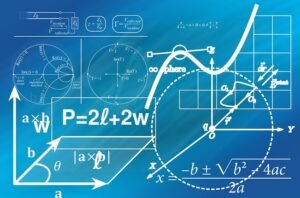# 5 Top Online University Maths Courses for StudentsPosted by

Maths, a vital subject that touches on every aspect of science, including chemistry and biology. Other commercial fields of study, such as economics, benefit from it as well. In fact, maths must be a part of our daily lives.

When you go to the store to buy groceries and other items, you’ll need to do some maths to figure out how much you’ve spent. Although the maths simple, it’s still maths. In the fields of engineering and construction, a lot of maths went into constructing that bridge or road you travel every day.As a result, we cannot ignore arithmetic; it is as vital as any other aspect of science. And you may acquire university maths courses from the comfort of your own house or any other location that seems convenient to you thanks to this post. Now is the time to grab your smartphone or computer.

After some digging, we have complied a list of top online university maths courses for students.

## Top Online Maths Courses for Students

Below is a list of top online university maths courses for students, attached are links to each course.

### 1. Geometry, Trigonometry, and Exponential

First on our list of top online university maths courses for students, is Geometry, Trigonometry, and Exponential.

You must have taken this arithmetic course in high school, nevertheless, this is a step forward from what you learned in high school.

Enrolling in this free online course will help you improve your calculus knowledge in the fields of geometry, trigonometry, and exponentials, as well as provide you with the abilities necessary to support further mathematical study.

### 2. Matrix Algebra for Engineers

If you are interested in learning matrix algebra, then this course in our list of top online university maths courses for students is for you.

Anyone interested in learning matrices, vector spaces, linear equations, and other topics can take the Matrix Algebra for Engineers course offered by the Hong Kong University of Science and Technology on Coursera.

You should enrol in this course if you are an engineering student or are still in high school and want to pursue an engineering course at a higher institution. It covers matrices and linear algebras, which are essential for engineers.

### 3. Mathematics for Economists

This course is required for future economists; it is an important aspect of the undergraduate stage of education for anyone interested in studying economics.

Mathematics for Economists is an online university maths course that provides students with the knowledge and abilities they need to solve mathematical issues in economics.

### 4. Advanced Statistics for Data Science Specialization

Advanced Statistics for Data Science Specialization is one of the top online university maths courses for students.

This course is one of the John Hopkins University’s online university maths courses, and it is taught by a John Hopkins University professor.

For Data Science, the course covers the principles of probability and statistics, data analysis, and linear models. Students will gain knowledge of biostatistics, R programming, linear regression, and other topics.### 5. Introduction to Complex Analysis

Introduction to Complex Analysis is one of Wesleyan University’s online university math courses available through Coursera. The course will teach you how to use power series, sophisticated analysis, mapping, and compiler optimization.

The first lesson begins with an introduction to complex analysis, which is the theory of complex functions of a complex variable and is free to enrol in. Then there’s an introduction to the complex plane, complex number algebra and geometry, integration, differentiation, and power series.

### 6. College Algebra

We end our list of top online university maths courses for students, with college algebra.

This course is designed for high school graduates who desire to continue their studies at a college or university level. You will study and learn numerous skills and knowledge in arithmetic, algebraic expressions, and equations, as well as use them to solve problems, when you enrol in this course.

Attempting college or university mathematics using your prior knowledge will be lot easier for you to grasp.